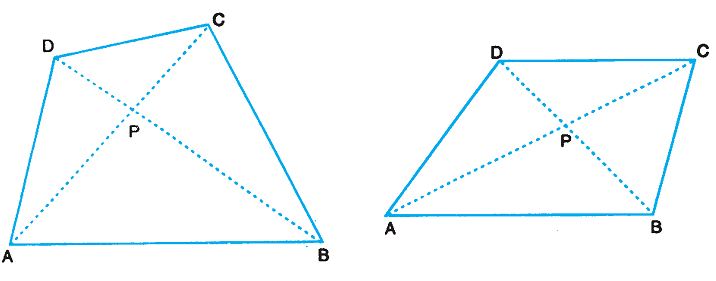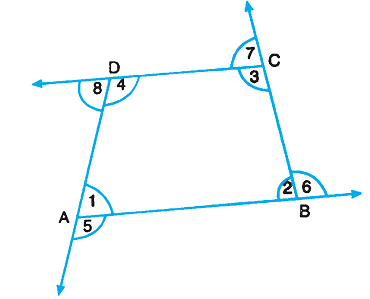If A, B, C and D are four points in a plane such that no three of them are collinear and the line segments AB, BC, CD and DA do not intersect except at their end points, then the closed figure made up of these four line segments is called a quadrilateral with vertices A, B, C and D.• AB and DC, BC and AD are two pairs of opposite sides.
• ∠A and ∠C, ∠B and ∠D are two pairs of opposite angles.
• AB and BC, BC and CD are two pairs of consecutive or adjacent sides.
• ∠A and ∠B, ∠B and ∠C are two pairs of consecutive or adjacent angles.
• AC and BD are the two diagonals.

Angles denoted by 1, 2, 3 and 4 are the interior angles or the angles of the quad ABCD. Angles denoted by 5, 6, 7 and 8 are the exterior angles of the quad ABCD.Sum of interior angles of a quadrilateral equals 360°.

Sum of exterior angles of a quadrilateral is also 360°.